# 16-bit table chip for SHA-256

This chip implementation is based around a single 16-bit lookup table. It requires a minimum of circuit rows, and is therefore suitable for use in larger circuits.

We target a maximum constraint degree of . That will allow us to handle constraining carries and "small pieces" to a range of up to in one row.

## Compression round

There are compression rounds. Each round takes 32-bit values as input, and performs the following operations:

where must handle a carry .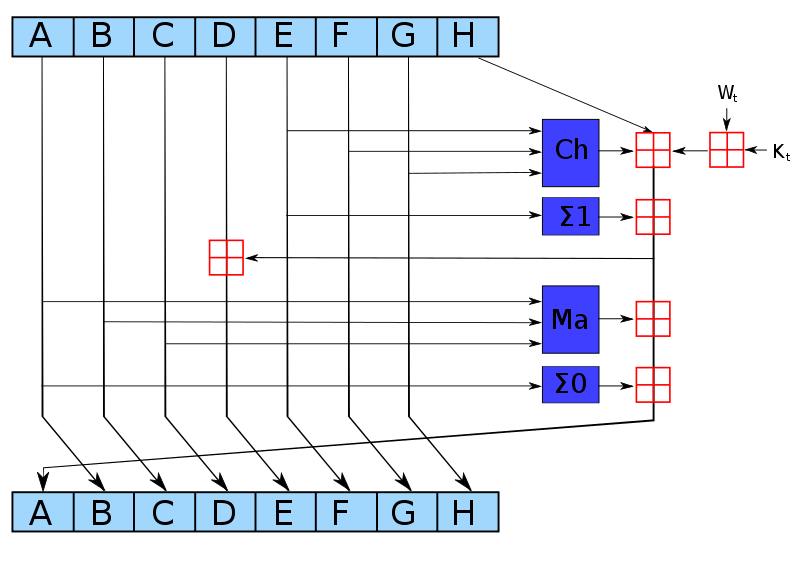Define as a table mapping a -bit input to an output interleaved with zero bits. We do not require a separate table for range checks because can be used.

To implement addition modulo , we note that this is equivalent to adding the operands using field addition, and then masking away all but the lowest 32 bits of the result. For example, if we have two operands and :

we decompose each operand (along with the result) into 16-bit chunks:

and then reformulate the constraint using field addition:

More generally, any bit-decomposition of the output can be used, not just a decomposition into 16-bit chunks. Note that this correctly handles the carry from .

This constraint requires that each chunk is correctly range-checked (or else an assignment could overflow the field).

• The operand and result chunks can be constrained using , by looking up each chunk in the "dense" column within a subset of the table. This way we additionally get the "spread" form of the output for free; in particular this is true for the output of the bottom-right which becomes , and the output of the leftmost which becomes . We will use this below to optimize and .

• must be constrained to the precise range of allowed carry values for the number of operands. We do this with a small range constraint.

### Maj function

can be done in lookups: chunks

• As mentioned above, after the first round we already have in spread form . Similarly, and are equal to the and respectively of the previous round, and therefore in the steady state we already have them in spread form and . In fact we can also assume we have them in spread form in the first round, either from the fixed IV or from the use of to reduce the output of the feedforward in the previous block.
• We can add them as -bit words or in pieces; it's equivalent
• Witness the compressed even bits and the compressed odd bits for ;
• Constrain , where is the function output.

Note: by "even" bits we mean the bits of weight an even-power of , i.e. of weight . Similarly by "odd" bits we mean the bits of weight an odd-power of .

### Ch function

TODO: can probably be optimized to or lookups using an additional table.

can be done in lookups: chunks

• As mentioned above, after the first round we already have in spread form . Similarly, and are equal to the and respectively of the previous round, and therefore in the steady state we already have them in spread form and . In fact we can also assume we have them in spread form in the first round, either from the fixed IV or from the use of to reduce the output of the feedforward in the previous block.
• Calculate and , where .
• We can add them as -bit words or in pieces; it's equivalent.
• works to compute the spread of even though negation and do not commute in general. It works because each spread bit in is subtracted from , so there are no borrows.
• Witness such that , and similarly for .
• is the function output.

### Σ_0 function

can be done in lookups.

To achieve this we first split into pieces , of lengths bits respectively counting from the little end. At the same time we obtain the spread forms of these pieces. This can all be done in two PLONK rows, because the and -bit pieces can be handled using lookups, and the -bit piece can be split into -bit subpieces. The latter and the remaining -bit piece can be range-checked by polynomial constraints in parallel with the two lookups, two small pieces in each row. The spread forms of these small pieces are found by interpolation.

Note that the splitting into pieces can be combined with the reduction of , i.e. no extra lookups are needed for the latter. In the last round we reduce after adding the feedforward (requiring a carry of up to which is fine).

is equivalent to :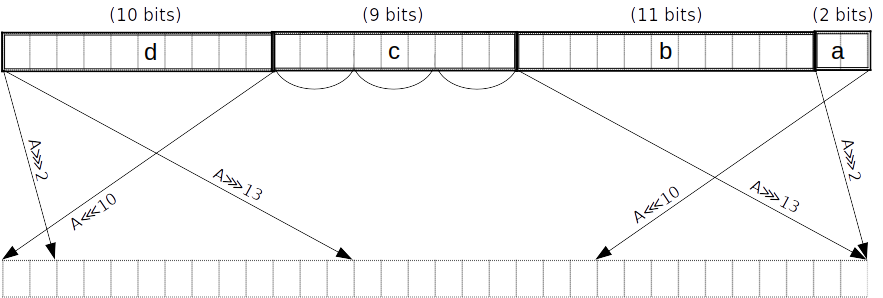Then, using more lookups we obtain the result as the even bits of a linear combination of the pieces:

That is, we witness the compressed even bits and the compressed odd bits , and constrain where is the function output.

### Σ_1 function

can be done in lookups.

To achieve this we first split into pieces , of lengths bits respectively counting from the little end. At the same time we obtain the spread forms of these pieces. This can all be done in two PLONK rows, because the and -bit pieces can be handled using lookups, the -bit piece can be split into and -bit subpieces, and the -bit piece can be split into -bit subpieces. The four small pieces can be range-checked by polynomial constraints in parallel with the two lookups, two small pieces in each row. The spread forms of these small pieces are found by interpolation.

Note that the splitting into pieces can be combined with the reduction of , i.e. no extra lookups are needed for the latter. In the last round we reduce after adding the feedforward (requiring a carry of up to which is fine).

is equivalent to .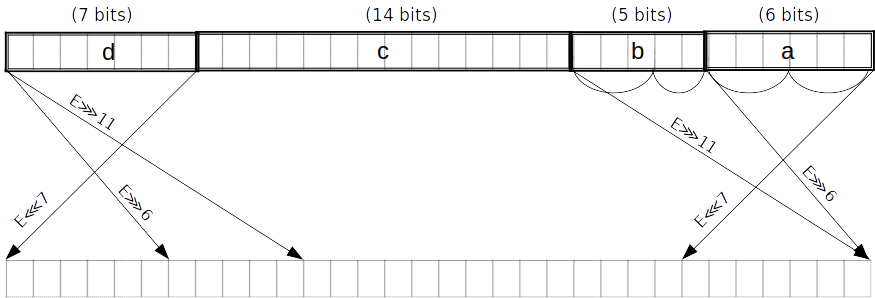Then, using more lookups we obtain the result as the even bits of a linear combination of the pieces, in the same way we did for :

That is, we witness the compressed even bits and the compressed odd bits , and constrain where is the function output.

## Block decomposition

For each block of the padded message, words of bits each are constructed as follows:

• The first are obtained by splitting into -bit blocks
• The remaining words are constructed using the formula: for .

Note: -based numbering is used for the word indices.

Note: is a right-shift, not a rotation.

### σ_0 function

is equivalent to .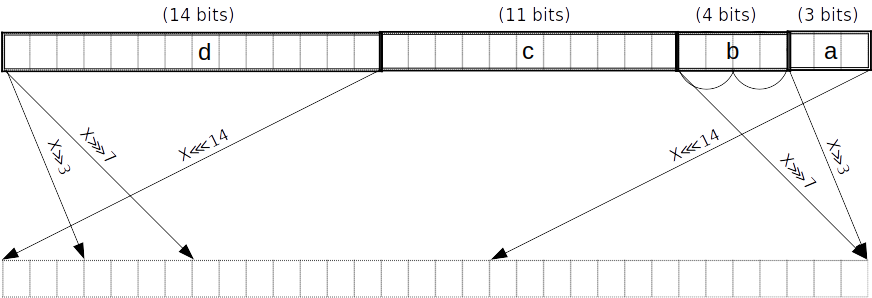As above but with pieces of lengths counting from the little end. Split into two -bit subpieces.

### σ_1 function

is equivalent to .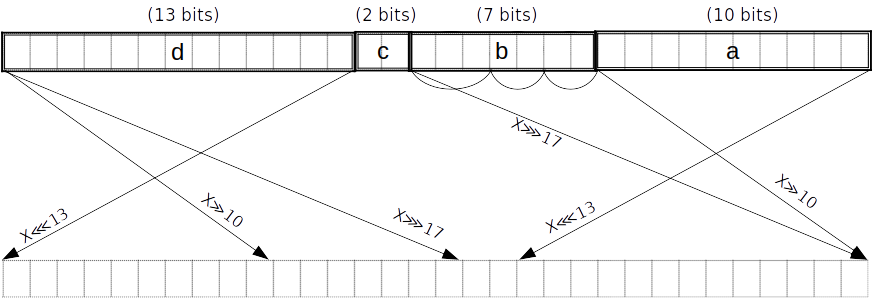TODO: this diagram doesn't match the expression on the right. This is just for consistency with the other diagrams.

As above but with pieces of lengths counting from the little end. Split into -bit subpieces.

### Message scheduling

We apply to , and to . In order to avoid redundant applications of , we can merge the splitting into pieces for and in the case of . Merging the piece lengths and gives pieces of lengths .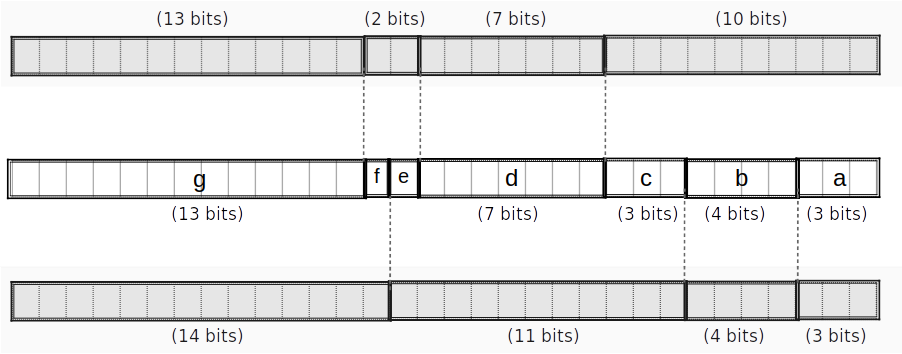If we can do the merged split in rows (as opposed to a total of rows when splitting for and separately), we save rows.

These might even be doable in rows; not sure. —Daira

We can merge the reduction mod of into their splitting when they are used to compute subsequent words, similarly to what we did for and in the round function.

We will still need to reduce since they are not split. (Technically we could leave them unreduced since they will be reduced later when they are used to compute and -- but that would require handling a carry of up to rather than , so it's not worth the complexity.)

The resulting message schedule cost is:

• rows to constrain to bits
• This is technically optional, but let's do it for robustness, since the rest of the input is constrained for free.
• rows to split into -bit pieces
• rows to split into -bit pieces (merged with a reduction for )
• rows to split into -bit pieces (merged with a reduction)
• rows to extract the results of for
• rows to extract the results of for
• rows to reduce
• rows.

## Overall cost

For each round:

• rows for
• rows for
• rows for
• rows for
• and are always free
• per round

This gives rows for all of "step 3", to which we need to add:

• rows for message scheduling
• rows for reductions mod in "step 4"

giving a total of rows.

## Tables

We only require one table , with rows and columns. We need a tag column to allow selecting -bit subsets of the table for and .

### spread table

0000000000000000000000000000000000000000000000000
0000000000000000100000000000000000000000000000001
0000000000000001000000000000000000000000000000100
0000000000000001100000000000000000000000000000101
...0......
0000000000111111100000000000000000001010101010101
1000000001000000000000000000000000100000000000000
...1......
1000000111111111100000000000001010101010101010101
...2......
2000001111111111100000000010101010101010101010101
...3......
3000111111111111100000001010101010101010101010101
...4......
4001111111111111100000101010101010101010101010101
...5......
5111111111111111101010101010101010101010101010101

For example, to do an -bit lookup, we polynomial-constrain the tag to be in . For the most common case of a -bit lookup, we don't need to constrain the tag. Note that we can fill any unused rows beyond with a duplicate entry, e.g. all-zeroes.

## Gates

### Choice gate

Input from previous operations:

• 64-bit spread forms of 32-bit words , assumed to be constrained by previous operations
• in practice, we'll have the spread forms of after they've been decomposed into 16-bit subpieces
• is defined as

s_ch
0{0,1,2,3,4,5}
1{0,1,2,3,4,5}
0{0,1,2,3,4,5}
0{0,1,2,3,4,5}

#### ¬E ∧ G

s_ch_neg
0{0,1,2,3,4,5}
1{0,1,2,3,4,5}
0{0,1,2,3,4,5}
0{0,1,2,3,4,5}

Constraints:

• s_ch (choice):
• s_ch_neg (negation): s_ch with an extra negation check
• lookup on
• permutation between

Output:

### Majority gate

Input from previous operations:

• 64-bit spread forms of 32-bit words , assumed to be constrained by previous operations
• in practice, we'll have the spread forms of after they've been decomposed into -bit subpieces
s_maj
0{0,1,2,3,4,5}
1{0,1,2,3,4,5}
0{0,1,2,3,4,5}
0{0,1,2,3,4,5}

Constraints:

• s_maj (majority):
• lookup on
• permutation between

Output:

### Σ_0 gate

is a 32-bit word split into -bit chunks, starting from the little end. We refer to these chunks as respectively, and further split into three 3-bit chunks . We witness the spread versions of the small chunks.

s_upp_sigma_0
0{0,1,2,3,4,5}
1{0,1,2,3,4,5}
0{0,1,2,3,4,5}
0{0,1,2,3,4,5}

Constraints:

• s_upp_sigma_0 ( constraint):

• lookup on
• 2-bit range check and 2-bit spread check on
• 3-bit range check and 3-bit spread check on

(see section Helper gates)

Output:

### Σ_1 gate

is a 32-bit word split into -bit chunks, starting from the little end. We refer to these chunks as respectively, and further split into two 3-bit chunks and into (2,3)-bit chunks . We witness the spread versions of the small chunks.

s_upp_sigma_1
0{0,1,2,3,4,5}
1{0,1,2,3,4,5}
0{0,1,2,3,4,5}
0{0,1,2,3,4,5}

Constraints:

• s_upp_sigma_1 ( constraint):

• lookup on
• 2-bit range check and 2-bit spread check on
• 3-bit range check and 3-bit spread check on

(see section Helper gates)

Output:

### σ_0 gate

#### v1

v1 of the gate takes in a word that's split into -bit chunks (already constrained by message scheduling). We refer to these chunks respectively as is further split into two 2-bit chunks We witness the spread versions of the small chunks. We already have and from the message scheduling.

is equivalent to .

s_low_sigma_0
0{0,1,2,3,4,5}
1{0,1,2,3,4,5}
0{0,1,2,3,4,5}
0{0,1,2,3,4,5}

Constraints:

• s_low_sigma_0 ( v1 constraint):

• check that b was properly split into subsections for 4-bit pieces.
• 2-bit range check and 2-bit spread check on
• 3-bit range check and 3-bit spread check on

#### v2

v2 of the gate takes in a word that's split into -bit chunks (already constrained by message scheduling). We refer to these chunks respectively as We already have from the message scheduling. The 1-bit remain unchanged by the spread operation and can be used directly. We further split into two 2-bit chunks We witness the spread versions of the small chunks.

is equivalent to .

s_low_sigma_0_v2
0{0,1,2,3,4,5}
1{0,1,2,3,4,5}
0{0,1,2,3,4,5}
0{0,1,2,3,4,5}

Constraints:

• s_low_sigma_0_v2 ( v2 constraint):

• check that b was properly split into subsections for 4-bit pieces.
• 2-bit range check and 2-bit spread check on
• 3-bit range check and 3-bit spread check on

### σ_1 gate

#### v1

v1 of the gate takes in a word that's split into -bit chunks (already constrained by message scheduling). We refer to these chunks respectively as is further split into -bit chunks We witness the spread versions of the small chunks. We already have and from the message scheduling.

is equivalent to .

s_low_sigma_1
0{0,1,2,3,4,5}
1{0,1,2,3,4,5}
0{0,1,2,3,4,5}
0{0,1,2,3,4,5}

Constraints:

• s_low_sigma_1 ( v1 constraint):

• check that b was properly split into subsections for 7-bit pieces.

• 2-bit range check and 2-bit spread check on

• 3-bit range check and 3-bit spread check on

#### v2

v2 of the gate takes in a word that's split into -bit chunks (already constrained by message scheduling). We refer to these chunks respectively as We already have from the message scheduling. The 1-bit remain unchanged by the spread operation and can be used directly. We further split into two 2-bit chunks We witness the spread versions of the small chunks.

is equivalent to .

s_low_sigma_1_v2
0{0,1,2,3,4,5}
1{0,1,2,3,4,5}
0{0,1,2,3,4,5}
0{0,1,2,3,4,5}

Constraints:

• s_low_sigma_1_v2 ( v2 constraint):

• check that b was properly split into subsections for 4-bit pieces.
• 2-bit range check and 2-bit spread check on
• 3-bit range check and 3-bit spread check on

### Helper gates

#### Small range constraints

Let . Constraining this expression to equal zero enforces that is in

#### 2-bit range check

sr2
1a

ss2
1aa'

with interpolation polynomials:

• ()
• ()
• ()
• ()

#### 3-bit range check

sr3
1a

ss3
1aa'

with interpolation polynomials:

• ()
• ()
• ()
• ()
• ()
• ()
• ()
• ()

#### reduce_6 gate

Input:

Check:

Assume inputs are constrained to 16 bits.

• Carry gate (sc):
sasc
10
11

Assume inputs are constrained to 16 bits.

• Carry gate (sc):
sasc
00
11
00
10